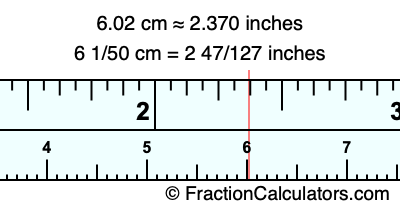6.02 cm in inchesHere is how to convert 6.02 cm to inches. We will give you the fractional answer, the decimal answer, and illustrate the answer on our tape measure.

One centimeter is equal to 50/127 inches, and 6.02 as a fraction is 6 1/50. Therefore, to get the fractional answer to 6.02 cm in inches, we multiply 6 1/50 by 50/127 and simplify if necessary.

cm × 50/127 = inches
6 1/50 × 50/127 = inches
6 1/50 × 50/127 = 2 47/127
6 1/50 cm = 2 47/127 inches
6.02 cm = 2 47/127 inches

As promised above, we also have the answer to 6.02 cm in inches in decimal form. Although the fractional answer above is exact, the decimal answer may be rounded if necessary:

2 47/127 ≈ 2.37007874015748
2 47/127 ≈ 2.370
6.02 cm ≈ 2.370 inches

Our image below shows 6.02 cm on a tape measure. The top row of the tape measure is inches, and the bottom row is centimeters.As you can see, we drew a red line where 6.02 cm and 2.370 inches meet on the measuring tape.

Centimeters to Inches Calculator
Here you can convert another length in centimeters to inches.

Convert  cm to inches.

What is 6.03 cm in inches?
Here is another inches to centimeters calculation we did for you.